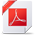# Decision Optimization

View Only

## Python_Docplex.CP#### Hugues JuilleMon May 02, 2022 09:06 AM#### Hugues JuilleMon May 02, 2022 09:13 AM#### Hugues JuilleTue May 03, 2022 08:53 AM#### Hugues JuilleWed May 04, 2022 04:22 AM• #### 1.  Python_Docplex.CP

Posted Mon May 02, 2022 05:36 AM

Hi,

In my code typeOfNext works in OPL but I have problem about adapting 'typeOfNext' in python, I would be glad if you give me idea!

Cplex_using CP:

tuple
triplet { int id1; int id2; int value; };

{triplet} M = { <i,j,d[i][j]> | i in nodes, j in nodes }

dvar sequence Q [v in w] in all (i in nodes)x[i][v]types all (i in nodes) i

dexpr int = sum (k in w,i in 0..nnodes) (d[i][typeOfNext(Q[k],x[i][k],i,i)]);

Python_docplex.CP:

------------------------------
------------------------------

Attachment(s)Python_DoCplex.pdf   62 KB 1 version

• #### 2.  RE: Python_Docplex.CP

Posted Mon May 02, 2022 09:06 AM
One issue comes from the definition of the Q[v] sequences.
These sequences should be defined as follows:

`Q={}`
`# for v in Vehicles:`
`#     for t in CustomersDepot:`
`#         Q[v]=mdl.sequence_var([x_i_k[(t, v)]], types=([t]))`
`#         print(Q[v])`

`for v in Vehicles:`
`    Q[v]=mdl.sequence_var([x_i_k[(t, v)] for t in CustomersDepot], types=([t for t in CustomersDepot]))`
`    print(Q[v])`

Following this change, the model can solve. However, there might be some other issues as the model is found infeasible...
Do you confirm a solution should be found with this model?
Best regards,
Hugues

------------------------------
Hugues Juille
------------------------------

• #### 3.  RE: Python_Docplex.CP

Posted Mon May 02, 2022 09:13 AM
Actually, running the conflict refiner on your model, the following conflict is returned:
`alternative(intervalVar(start=95, end=125, size=10), [intervalVar(optional, start=95, end=125, size=10), intervalVar(optional, start=95, end=125, size=10), intervalVar(optional, start=95, end=125, size=10)])`

In fact, there is a problme in the definition of your task intervals since there are all infeasible (if you do want to define: `start`, `end` and `size`, then one should have: `end = start + size`)

Best regards,
Hugues

------------------------------
Hugues Juille
------------------------------

• #### 4.  RE: Python_Docplex.CP

Posted Mon May 02, 2022 04:17 PM

Dear Hugues,

Thanks a lot for the reply.
In fact my code works in OPL correctly. I attached it and you can take a short look on it.

Best regards,

------------------------------
------------------------------

• #### 5.  RE: Python_Docplex.CP

Posted Tue May 03, 2022 08:53 AM
Edited by Hugues Juille Tue May 03, 2022 08:56 AM
Thanks for sharing your OPL model (but I would have preferred a text version :-) ).
In your python model, the `start` and `end` bounds for interval variables `x_i_k` and `y_i` are not defined properly.
You should replace their definition as follows:
`iv=mdl.interval_var(start=(ReadyTime[t], INTERVAL_MAX), end=(0, DueTime[t]), size=ser[t])`
and
`y_i[t]=mdl.interval_var(start=(ReadyTime[t], INTERVAL_MAX), end=(0, DueTime[t]), size=ser[t])`
`start` and `end` are pairs of values that define the bounds for the interval (the documentation can be found here: https://rawgit.com/IBMDecisionOptimization/docplex-doc/master/docs/cp/docplex.cp.expression.py.html#docplex.cp.expression.interval_var).
After making that changes, the python model find an optimal solution.

Best regards,

Hugues

------------------------------
Hugues Juille
------------------------------

• #### 6.  RE: Python_Docplex.CP

Posted Tue May 03, 2022 01:39 PM
Dear Hugues,

I really appreciate for the reply.

Yes you are right, after changing the mentioned ones, that part of model works but it shows another error in no overlap constraint and most probably typeOfNext is incorrect as well (attached the file)! I should derive the d(i)(j) parameter from my distance matrix and the second index value (j) should be entered as the typeofnext function to be able to derive the next customer, the vehicle visits after customer but sadly I can not code it properly!  I would be really glad if you check these parts too. As already I mentioned my code in OPLE works correctly and I can share text version if you need. I think problems are just adopting nooverlap and typeofnext.

Best regards,

------------------------------
------------------------------

Attachment(s)CP_Python.pdf   64 KB 1 version

• #### 7.  RE: Python_Docplex.CP

Posted Wed May 04, 2022 04:22 AM
I found a few more issues in the python model that I overlooked...
First: constraints need to be added explicitly to the model. This applies to the following statements (where `mdl.add(...)` was initially missing):
`for v in Vehicles:`
`    mdl.add(mdl.no_overlap(Q[v], distance_matrix))`
`    # mdl.add(mdl.no_overlap(Q[v]))`
and:
`for v in Vehicles:`
`    mdl.add(mdl.presence_of(x_i_k[(0,v)])==1)`
`    mdl.add(mdl.presence_of(x_i_k[(11,v)])==1)`
`    mdl.add(mdl.first(Q[v], x_i_k[(0,v)]))`
`    mdl.add(mdl.last(Q[v], x_i_k[(11,v)]))`

Then, I'm not sure to follow your formulation for the objective. I think the following formulation is expected:
`# obj=sum((TravelDistance[t, t] * mdl.type_of_next(Q[v], x_i_k[(t, v)], t, t)) for v in Vehicles for t in CustomersDepot)`
`obj=sum((mdl.element([TravelDistance[t, t_dest] for t_dest in CustomersDepot], mdl.type_of_next(Q[v], x_i_k[(t, v)], t, t)) for v in Vehicles for t in CustomersDepot))`

Unfortunately, after implementing these changes the model is unfeasible...
If the distance matrix in the `no_overlap` constraint is removed, the model becomes feasible.
I'm afraid there may be some data issues in the distance matrix or the start/end for intervals that makes the model unfeasible.
If you have a working OPL model, you should try to compare these data to understand where the difference is coming from...

Also, use text format when sharing models, if possible. That would make things much easier on my side to try to replicate your issues :-)
Best regards,

Hugues

------------------------------
Hugues Juille
------------------------------

• #### 8.  RE: Python_Docplex.CP

Posted Wed May 11, 2022 12:31 PM
Hi Hugues,

I am really thankful to the reply.
You are right, after changing the mentioned ones my model works and for noOverlap part there is no need distance matrix! model is feasible and also near to optimal solution just there is a small problem about cumulFanction, this function works correctly for pick up delivery in our model but for simultaneous pickup delivery I think it is not correct and I have to  change cumulFanction but i do not have idea.
I would be glad if you give me some hints how to code this part and check and revise cumulFanction constraint

Best regards,